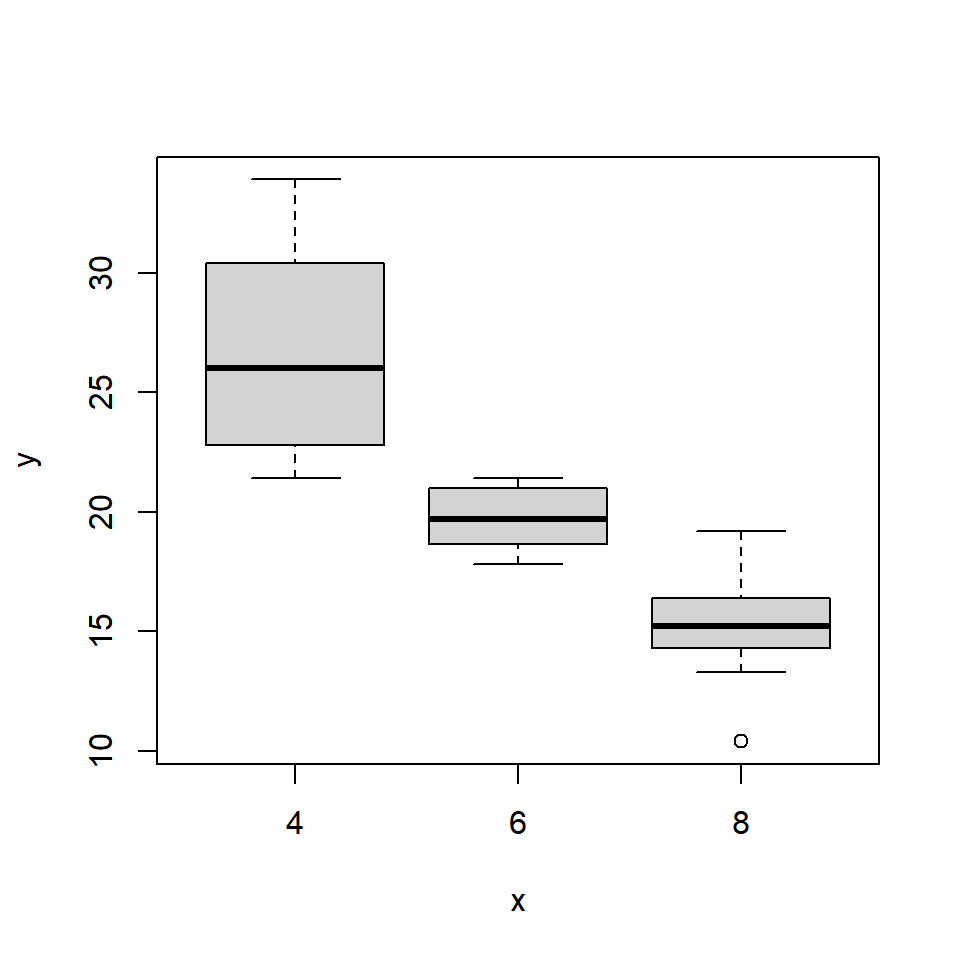# Chapter 1 Introduction

## 1.1 Introduction

This is the first post of the series Data Visualization With R. The objective of the series is to provide a gentle introduction to working with base graphics in R. We will come up with a similar series using ggplot2 shortly.

• what is data visualization
• why visualize data
• understand R graphics system
• graphics
• ggplot2
• lattice
• build some simple plots

## 1.2 What is data visualization?

In simple words, data visualization is the representation of data in graphical format.data-viz

## 1.3 Why visualize data?

• Explore: Visualization helps in exploring and explaining patterns and trends
• Detect: Patterns or anomalies in data can be detected by looking at graphs
• Make sense: Possible to make sense of large amount of data efficiently and in time
• Communicate: Easy to communicate and share the insights from data

## 1.4 R Graphics System

• graphics
• ggplot2
• lattice

### 1.4.1 Graphics

• It is part of base R and is the fundamental package for visualizing data.
• It has a lot of good features and we can create all the basic plots using it.

### 1.4.2 ggplot2

ggplot2, created by Hadley Wickham, is based on the Grammar of Graphics written by Leland Wilkinson. It has a structured approach to data visualization and builds upon the features available in the Graphics and Lattice packages.

### 1.4.3 Lattice

The lattice package is inspired by Trellis Graphics and created by Deepayan Sarkar. It is a very powerful data visualization system with an emphasis on multivariate data.

## 1.5 Getting Help

Use the `help()` to learn more about `plot()` function and `mtcars` data set.

``````help(plot)
help(mtcars)``````

### 1.5.1 mtcars

``head(mtcars)``
``````##                    mpg cyl disp  hp drat    wt  qsec vs am gear carb
## Mazda RX4         21.0   6  160 110 3.90 2.620 16.46  0  1    4    4
## Mazda RX4 Wag     21.0   6  160 110 3.90 2.875 17.02  0  1    4    4
## Datsun 710        22.8   4  108  93 3.85 2.320 18.61  1  1    4    1
## Hornet 4 Drive    21.4   6  258 110 3.08 3.215 19.44  1  0    3    1
## Hornet Sportabout 18.7   8  360 175 3.15 3.440 17.02  0  0    3    2
## Valiant           18.1   6  225 105 2.76 3.460 20.22  1  0    3    1``````

### 1.5.2 Variable Info

``str(mtcars)``
``````## 'data.frame':    32 obs. of  11 variables:
##  \$ mpg : num  21 21 22.8 21.4 18.7 18.1 14.3 24.4 22.8 19.2 ...
##  \$ cyl : num  6 6 4 6 8 6 8 4 4 6 ...
##  \$ disp: num  160 160 108 258 360 ...
##  \$ hp  : num  110 110 93 110 175 105 245 62 95 123 ...
##  \$ drat: num  3.9 3.9 3.85 3.08 3.15 2.76 3.21 3.69 3.92 3.92 ...
##  \$ wt  : num  2.62 2.88 2.32 3.21 3.44 ...
##  \$ qsec: num  16.5 17 18.6 19.4 17 ...
##  \$ vs  : num  0 0 1 1 0 1 0 1 1 1 ...
##  \$ am  : num  1 1 1 0 0 0 0 0 0 0 ...
##  \$ gear: num  4 4 4 3 3 3 3 4 4 4 ...
##  \$ carb: num  4 4 1 1 2 1 4 2 2 4 ...``````

## 1.6 plot()

Now that we have some idea about the data set, let us explore the `plot()` function. We will use the following different data inputs and observe the kind of plots that are generated:

• Case 1: 1 continuous variable
• Case 2: 1 categorical variable
• Case 3: 2 continuous variables
• Case 4: 2 categorical variables
• Case 5: 1 continuous and 1 categorical variable
• Case 6: 1 categorical and 1 continuous variable

### 1.6.1 One continuous variable

``plot(mtcars\$mpg)``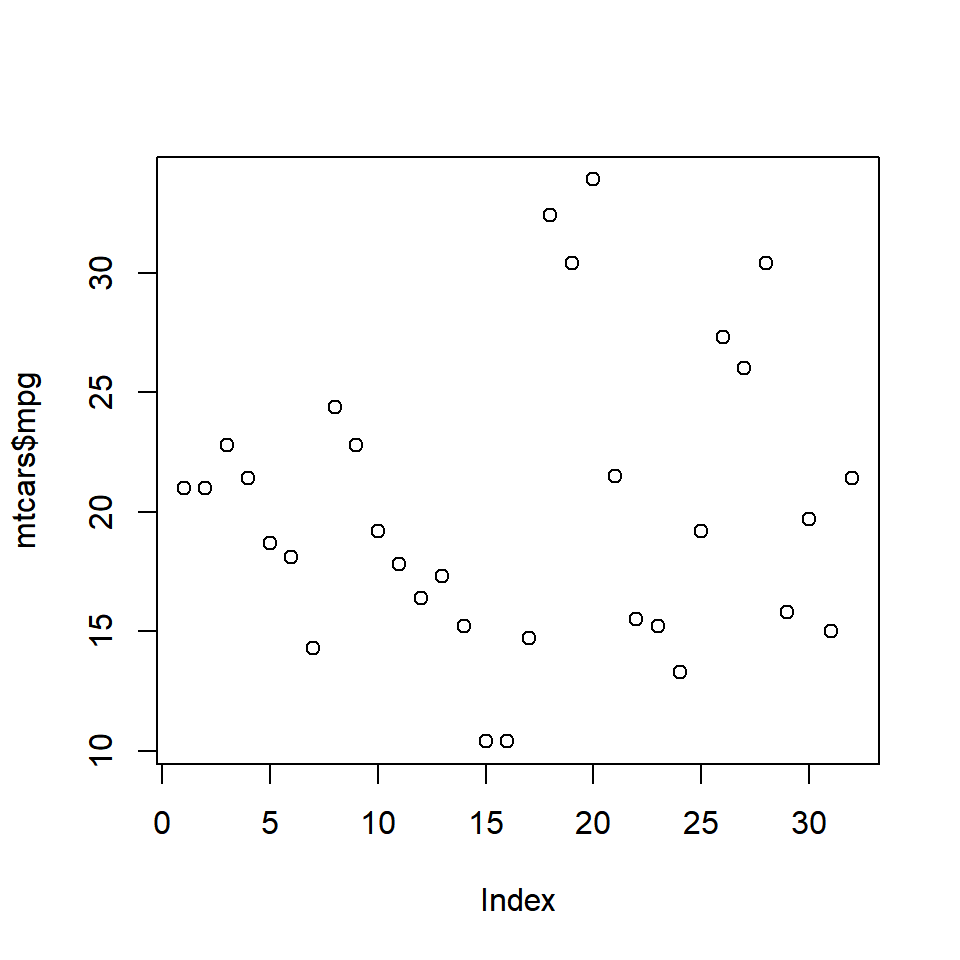### 1.6.2 One categorical variable

``plot(as.factor(mtcars\$cyl))``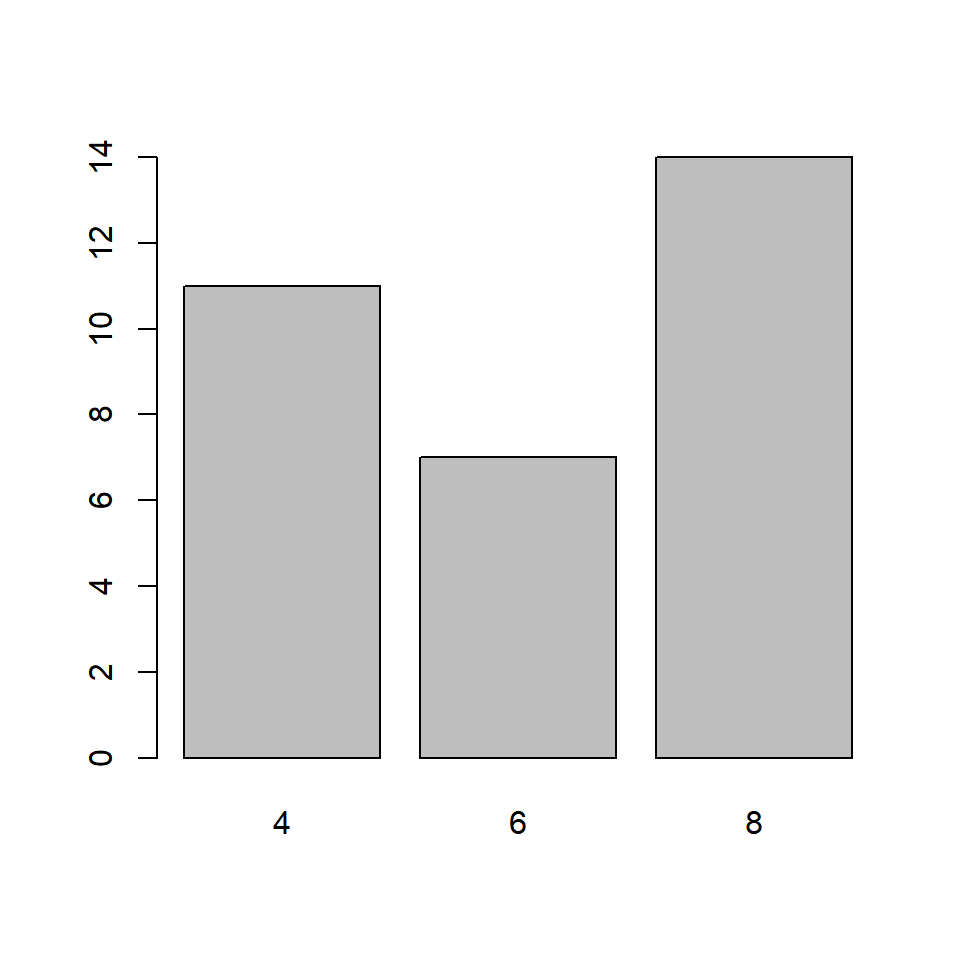### 1.6.3 Two continuous variables

``plot(mtcars\$disp, mtcars\$mpg)``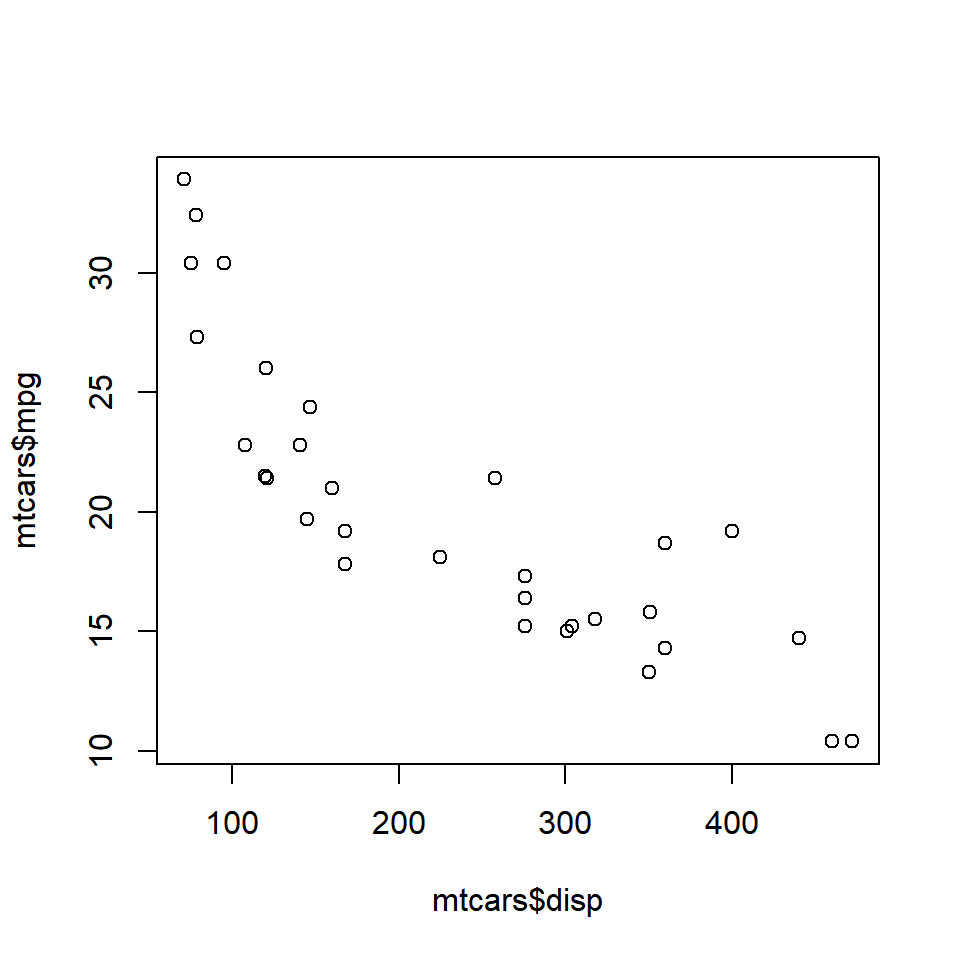### 1.6.4 Two categorical variables

``plot(as.factor(mtcars\$am), as.factor(mtcars\$cyl))``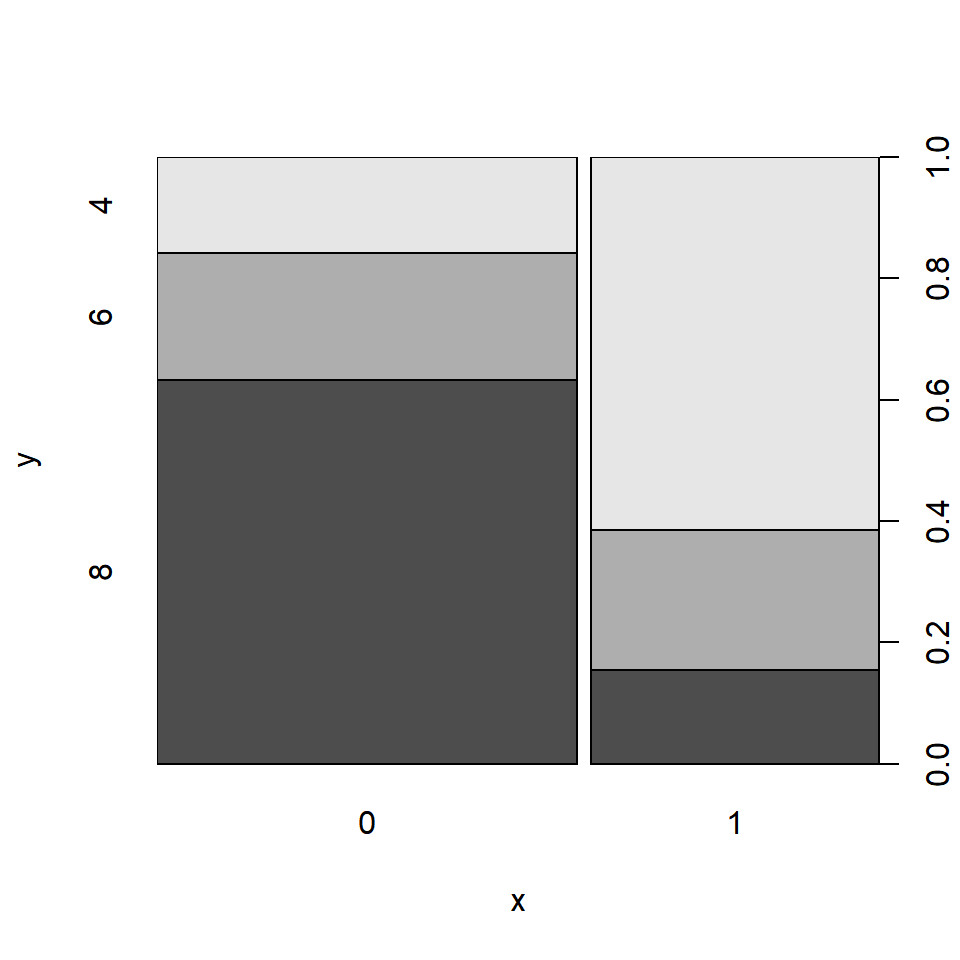### 1.6.5 Continuous/Categorical variable

``plot(mtcars\$mpg, mtcars\$cyl)``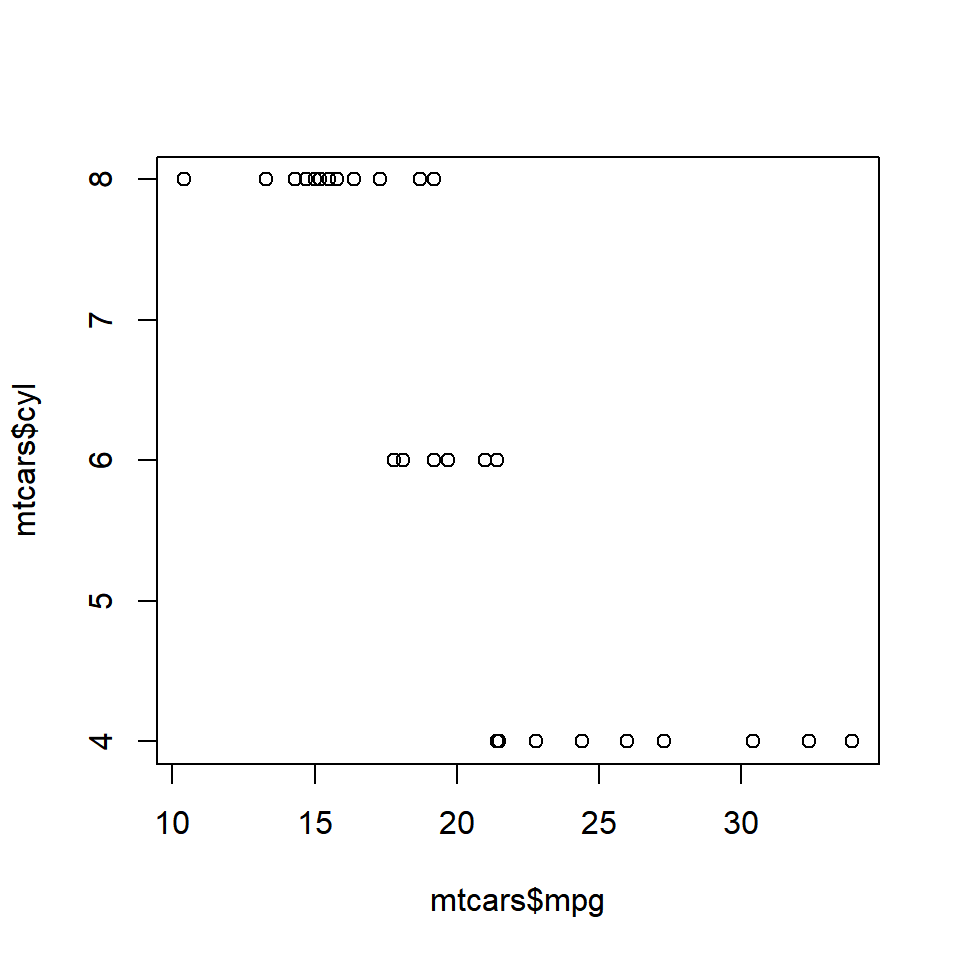### 1.6.6 Categorical/Continuous variable

``plot(as.factor(mtcars\$cyl), mtcars\$mpg)``